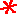Main      Site Guide### Scrambled Equations

• Scrambled Equations

In each of these puzzles, you are given a number that you must construct out of several other numbers. You do this by taking the numbers and performing addition, subtraction, multiplication, and/or division operations on them. Each number must be used in the calculations exactly once, and only these four operations listed may be used. You may parenthesize your expression however you wish. For example, 5 may be obtained from 1, 2, and 3, with the expression (3 + 2) × 1.

In many if not most cases, multiple solutions are possible, but usually only one is given on the solution page.

## #126

Make 15 out of 1, 5, 7, and 8.

Hint

Solution

## #127Make 32 out of 1, 3, 4, and 8.

Hint

Solution

## #128Make 5 out of 1, 4, 5, and 9.

Hint

Solution

## #129Make 9 out of 2, 2, 5, and 7.

Hint

Solution

## #130Make 14 out of 2, 2, 5, and 7.

Hint

Solution

## #131

Make 44 out of 1, 2, 7, and 9.

Hint

Solution

## #132

Make 26 out of 2, 5, 4, and 7.

Hint

Solution

## #133

Make 38 out of 1, 2, 4, and 5.

Hint

Solution

## #134

Make 36 out of 4, 6, 9, and 9.

Hint

Solution

## #135

Make 17 out of 2, 2, 6, and 6.

Hint

Solution

## #136Make 45 out of 2, 4, 6, and 8.

Hint

Solution

## #137

Make 20 out of 1, 6, 7, and 9.

Hint

Solution

## #138

Make 64 out of 3, 4, 5, and 7.

Hint

Solution

## #139

Make 46 out of 1, 2, 6, and 9.

Hint

Solution

## #140Make 26 out of 2, 3, 6, and 9.

Hint

Solution

## #141Make 44 out of 3, 5, 6, and 9.

Hint

Solution